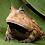### Find minimum oriented bounding box of point cloud (C++ and PCL)

Here we're trying to get the minimum oriented bounding box of a point cloud using C++ and the Point Cloud Library (PCL). Most of the code originates from user Nicola Fioraio on the PCL forums in this post.

Here is how user Nicola Fioraio describes the process:
1) compute the centroid (c0, c1, c2) and the normalized covariance
2) compute the eigenvectors e0, e1, e2. The reference system will be (e0, e1, e0 X e1) --- note: e0 X e1 = +/- e2
3) move the points in that RF --- note: the transformation given by the rotation matrix (e0, e1, e0 X e1) & (c0, c1, c2) must be inverted
4) compute the max, the min and the center of the diagonal
5) given a box centered at the origin with size (max_pt.x - min_pt.x, max_pt.y - min_pt.y, max_pt.z - min_pt.z) the transformation you have to apply is Rotation = (e0, e1, e0 X e1) & Translation = Rotation * center_diag + (c0, c1, c2)

And here is my interpretation of the process along with the code. Here's the cloud we're starting with (it's a sorghum plant):

As I understand it, we first find the eigenvectors for the covariance matrix of the point cloud (i.e. principal component analysis, PCA). You'll replace the cloudSegmented pointer with a pointer to the cloud you want to find the oriented bounding box for.
```// Compute principal directions
Eigen::Vector4f pcaCentroid;
pcl::compute3DCentroid(*cloudSegmented, pcaCentroid);
Eigen::Matrix3f covariance;
computeCovarianceMatrixNormalized(*cloudSegmented, pcaCentroid, covariance);
Eigen::Matrix3f eigenVectorsPCA = eigen_solver.eigenvectors();
eigenVectorsPCA.col(2) = eigenVectorsPCA.col(0).cross(eigenVectorsPCA.col(1));  /// This line is necessary for proper orientation in some cases. The numbers come out the same without it, but
///    the signs are different and the box doesn't get correctly oriented in some cases.
/* // Note that getting the eigenvectors can also be obtained via the PCL PCA interface with something like:
pcl::PointCloud<pcl::PointXYZ>::Ptr cloudPCAprojection (new pcl::PointCloud<pcl::PointXYZ>);
pcl::PCA<pcl::PointXYZ> pca;
pca.setInputCloud(cloudSegmented);
pca.project(*cloudSegmented, *cloudPCAprojection);
std::cerr << std::endl << "EigenVectors: " << pca.getEigenVectors() << std::endl;
std::cerr << std::endl << "EigenValues: " << pca.getEigenValues() << std::endl;
// In this case, pca.getEigenVectors() gives similar eigenVectors to eigenVectorsPCA.
*/
```
These eigenvectors are used to transform the point cloud to the origin point (0, 0, 0) such that the eigenvectors correspond to the axes of the space. The minimum point, maximum point, and the middle of the diagonal between these two points are calculated for the transformed cloud (also referred to as the projected cloud when using PCL's PCA interface, or reference cloud by Nicola).
```// Transform the original cloud to the origin where the principal components correspond to the axes.
Eigen::Matrix4f projectionTransform(Eigen::Matrix4f::Identity());
projectionTransform.block<3,3>(0,0) = eigenVectorsPCA.transpose();
projectionTransform.block<3,1>(0,3) = -1.f * (projectionTransform.block<3,3>(0,0) * pcaCentroid.head<3>());
pcl::PointCloud<pcl::PointXYZ>::Ptr cloudPointsProjected (new pcl::PointCloud<pcl::PointXYZ>);
pcl::transformPointCloud(*cloudSegmented, *cloudPointsProjected, projectionTransform);
// Get the minimum and maximum points of the transformed cloud.
pcl::PointXYZ minPoint, maxPoint;
pcl::getMinMax3D(*cloudPointsProjected, minPoint, maxPoint);
const Eigen::Vector3f meanDiagonal = 0.5f*(maxPoint.getVector3fMap() + minPoint.getVector3fMap());
```
This gives us something like this; the orange cloud is the transformed cloud (shown with an axis-aligned bounding box, but we want the oriented bounding box so we're not done yet).

Finally, the quaternion is calculated using the eigenvectors (which determines how the final box gets rotated), and the transform to put the box in correct location is calculated. The minimum and maximum points are used to determine the box width, height, and depth.
```// Final transform
const Eigen::Quaternionf bboxQuaternion(eigenVectorsPCA); //Quaternions are a way to do rotations https://www.youtube.com/watch?v=mHVwd8gYLnI
const Eigen::Vector3f bboxTransform = eigenVectorsPCA * meanDiagonal + pcaCentroid.head<3>();

// This viewer has 4 windows, but is only showing images in one of them as written here.
pcl::visualization::PCLVisualizer *visu;
visu = new pcl::visualization::PCLVisualizer (argc, argv, "PlyViewer");
int mesh_vp_1, mesh_vp_2, mesh_vp_3, mesh_vp_4;
visu->createViewPort (0.0, 0.5, 0.5, 1.0,  mesh_vp_1);
visu->createViewPort (0.5, 0.5, 1.0, 1.0,  mesh_vp_2);
visu->createViewPort (0.0, 0, 0.5, 0.5,  mesh_vp_3);
visu->createViewPort (0.5, 0, 1.0, 0.5, mesh_vp_4);
visu->addPointCloud(cloudSegmented, ColorHandlerXYZ(cloudSegmented, 30, 144, 255), "bboxedCloud", mesh_vp_3);
visu->addCube(bboxTransform, bboxQuaternion, maxPoint.x - minPoint.x, maxPoint.y - minPoint.y, maxPoint.z - minPoint.z, "bbox", mesh_vp_3);
```
And the final product is the bounding box around the original blue cloud.

1.i got some error,‘transformPointCloud’ is not a member of ‘pcl’ can you tell me what are the header file should i include?

1.I think it is part of transforms.hpp in the common module (source: http://docs.pointclouds.org/trunk/group__common.html and http://docs.pointclouds.org/trunk/transforms_8hpp_source.html )

Something like

#include <pcl/common/transforms.h>

should be sufficient (though I haven't tested it).

2.hey this is amazing,great algorithm.very smart man.keep it.i wish u all the best for your future works

2.Hi!
I have two carton boxes moving on a conveyor. After segmentation, there are two point clouds each belonging to a carton box. Can i fit a "bounding box" to the point clouds of these carton boxes at the same time and measure the dimensions of the boxes in real time?

1.That sounds like something that should be possible in real time.